Get inspired by the success stories of our students in IIT JAM MS, ISI  MStat, CMI MSc DS.  Learn More

# Vandermone's SRSWR | MStat 2017 PSB Problem 3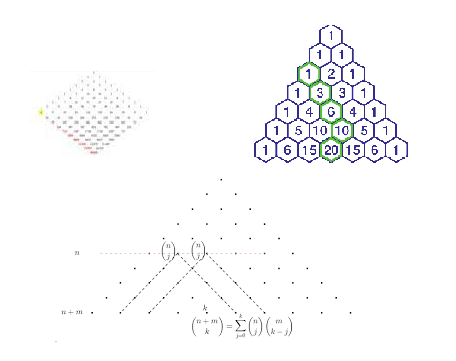This is a beautiful problem form ISI MStat 2017 PSB Problem 3, where we use the basics of Bijection principle and Vandermone's identity to solve this problem.

## Problem

Consider an urn containing 5 red, 5 black, and 10 white balls. If balls are drawn without replacement from the urn, calculate the probability that in the first 7 draws, at least one ball of each color is drawn.

## Prerequisites

• Algebrify the problem
• Vandermone's Identity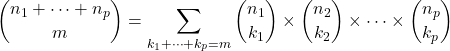## Solution

It may give you the intuition, there is atleast in the problem, so let's do complementing counting. Okay! I suggest you to travel that path to help you realize the complexity of that approach.

Nevertheless, let's algebrify the problem.

You want to select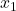red balls,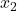blue balls, and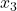white balls so that.

Now,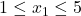,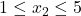and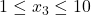represents our desired scenario.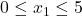,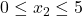and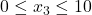denotes total number of cases.

Now, for each such triplet (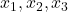) of the number of balls of each colour we have selected, we can select them in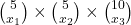.

Let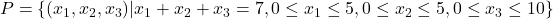.

Total Number of Ways we can select the 7 balls =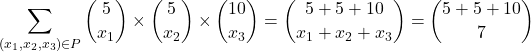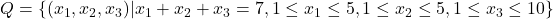.

Total Number of Ways we can select the 7 balls such that at least one ball of each color is drawn =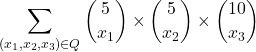Let,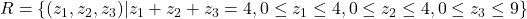.

Observe thathas a bijection with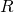.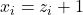.

Total Number of Ways we can select the 7 balls such that at least one ball of each color is drawn =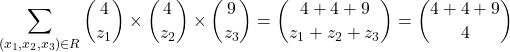## Exercises

• Prove the Vandermone's Identity.
• Find the Probability.
• Generalize the problem to general numbers and prove it.
• Generalize the problem where the lower bounds ofare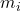.
• What if there are upper bounds on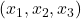? [ Hint: Generating Functions ].

Stay Tuned! Stay Blessed!

This is a beautiful problem form ISI MStat 2017 PSB Problem 3, where we use the basics of Bijection principle and Vandermone's identity to solve this problem.

## Problem

Consider an urn containing 5 red, 5 black, and 10 white balls. If balls are drawn without replacement from the urn, calculate the probability that in the first 7 draws, at least one ball of each color is drawn.

## Prerequisites

• Algebrify the problem
• Vandermone's Identity## Solution

It may give you the intuition, there is atleast in the problem, so let's do complementing counting. Okay! I suggest you to travel that path to help you realize the complexity of that approach.

Nevertheless, let's algebrify the problem.

You want to selectred balls,blue balls, andwhite balls so that.

Now,,andrepresents our desired scenario.,anddenotes total number of cases.

Now, for each such triplet () of the number of balls of each colour we have selected, we can select them in.

Let.

Total Number of Ways we can select the 7 balls =.

Total Number of Ways we can select the 7 balls such that at least one ball of each color is drawn =Let,.

Observe thathas a bijection with..

Total Number of Ways we can select the 7 balls such that at least one ball of each color is drawn =## Exercises

• Prove the Vandermone's Identity.
• Find the Probability.
• Generalize the problem to general numbers and prove it.
• Generalize the problem where the lower bounds ofare.
• What if there are upper bounds on? [ Hint: Generating Functions ].

Stay Tuned! Stay Blessed!

This site uses Akismet to reduce spam. Learn how your comment data is processed.

### Knowledge Partner